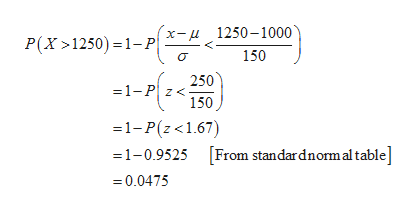# A lightbulb is advertised as lasting 1000 hours with a standard deviation of 150 hours. Assume the "life" of a lightbulb is normally distributed. determine the probability that a randomly selected lightbulb will last MORE than 1250 hours

Question
6 views

A lightbulb is advertised as lasting 1000 hours with a standard deviation of 150 hours. Assume the "life" of a lightbulb is normally distributed.

determine the probability that a randomly selected lightbulb will last MORE than 1250 hours

check_circle

Step 1

It is given that, the mean and standard deviation are 1,000 and 150, respectively.

Step 2

The probability that a randomly selected light bulb will ...help_outlineImage Transcriptionclosex-u1250-1000 150 P(X1250) 1-P 250 =1-P z< 150 =1-P(z<1.67) [From standar dnormal table] =1-0.9525 0.0475 fullscreen

### Want to see the full answer?

See Solution

#### Want to see this answer and more?

Solutions are written by subject experts who are available 24/7. Questions are typically answered within 1 hour.*

See Solution
*Response times may vary by subject and question.
Tagged in

### Other